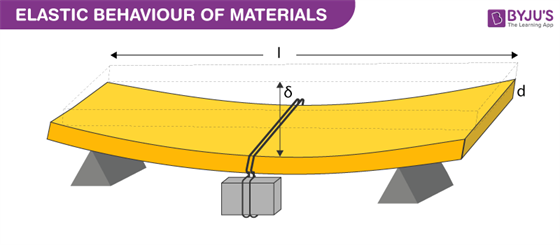# Elastic Behaviour Of Materials

What happens to a slingshot when you stretch it? It deforms but regains its original shape when you stop applying a force. But let us say that you take a thin steel rod and try to bend. You manage to bend it a little and then stop applying force. Does the rod regain its original shape? No, it doesn’t. This difference in the behaviour of the material is based on their elastic and plastic nature which is explained using Hooke’s law.The rubber strip of the slingshot has high elasticity. Elasticity is the ability of a body to resist any permanent change to it when stress is applied. When stress application ceases, the body regains its original shape and size. Different materials show different elastic behavior. The study of the elastic behaviour of a material is of much importance. Almost every engineering design requires knowledge of the elastic behaviour of materials. In the construction of various structures like bridges, columns, pillars, beams, etc. knowledge of the strength of the materials used in the construction is of prime importance.

For example: while constructing a bridge, a load of traffic that it can withstand should be adequately measured beforehand. Or while constructing a crane used to lift loads, it is kept in mind that the extension of the rope does not exceed the elastic limit of the rope. To overcome the problem of bending under force the elastic behaviour of the material used must be considered primarily.

To study the elastic behaviour of materials let us consider a beam of length l, breadth b and depth d supported at the ends and loaded at the centre by load W.In this case, it is given as;

$$\begin{array}{l}\delta = \frac{Wl^{3}}{4bd^{3}Y}\end{array}$$

Where,
δ is the sag
Y is Young’s modulus of elasticity

Using the above equation we can easily say that to reduce the amount of bending for a certain load, Young’s modulus of elasticity of the material used must be large. Also, depth d must be considered since sag is inversely proportional to the cube of depth. But the problem faced with increasing the depth is that bending increases and this is known as buckling. Therefore, a compromise is made between the different cross-sectional shapes.

### Recommended VideoTest Your Knowledge On Elastic Behaviour Of Materials!